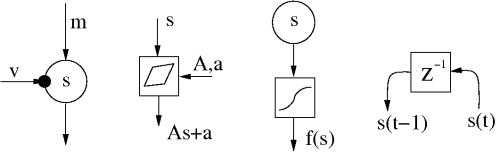# Building blocks for variational Bayesian learning

In graphical models, there are lots of possibilities to build model structures that define the dependencies between the parameters and the data. To be able to manage the variety, we have designed a modular software package using C++/Python called Bayes Blocks.

The design principles and theoretical background for the Bayes Blocks, as well as some application examples have been presented fairly thoroughly in the long journal paper (Raiko et al., 2007). The design principles are the following. First, we use standardized building blocks that can be connected rather freely and can be learned with local learning rules. Second, the system should work with very large scale models. We have made the computational complexity linear with respect to the number of data samples and connections in the model.

The building blocks include Gaussian variables, summation, multiplication, and nonlinearity. Recently, several new blocks were implemented including mixture-of-Gaussians and rectified Gaussians (Harva et al., 2005). Each of the blocks can be a scalar or a vector. Variational Bayesian learning provides a cost function which can be used for updating the variables as well as optimizing the model structure. The derivation of the cost function and learning rules is automatic, which means that the user only needs to define the connections between the blocks.

Examples of structures which can be build using the Bayes Blocks library can be found in (Raiko et al., 2007; Harva et al., 2005; Valpola et al., 2004), as well as in the seminal conference paper (Valpola et al., 2001) in which the blocks approach was introduced already in 2001.The Bayes Blocks include Gaussian variables with a mean and a variance inputs, addition and multiplications that can be used to form linear mappings, nonlinearities, and delays used for dynamic modelling.

### References

M. Harva, T. Raiko, A. Honkela, H. Valpola, and J. Karhunen, "Bayes blocks: An implementation of the variational Bayesian building blocks Framework." In Proc. of the 21st Conf. on Uncertainty in Artificial Intelligence (UAI 2005), Edinburgh, Scotland, 2005, pp. 259-266.
Pdf (164k)

T. Raiko, H. Valpola, M. Harva, and J. Karhunen, "Building blocks for variational Bayesian learning of latent variable models". In the Journal of Machine Learning Research (JMLR), volume 8, pages 155-201, January, 2007. Publisher electronic edition.

H. Valpola, T. Raiko, and J. Karhunen, "Building blocks for hierarchical latent variable models". In Proc. of the 3rd Int. Conf. on Independent Component Analysis and Signal Separation (ICA2001), San Diego, USA, 2001, pp. 710-715. Pdf (99k).

H. Valpola, M. Harva, and J. Karhunen, "Hierarchical models of variance sources". Signal Processing vol. 84, no. 2, 2004, pp. 267-282. Pdf (1128k)

You are at: CISResearchAlgorithmsBayes → Research

Page maintained by juha.karhunen at hut.fi, last updated Wednesday, 01-Oct-2008 10:28:43 EEST